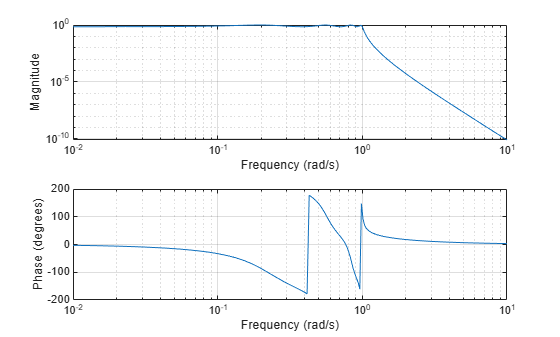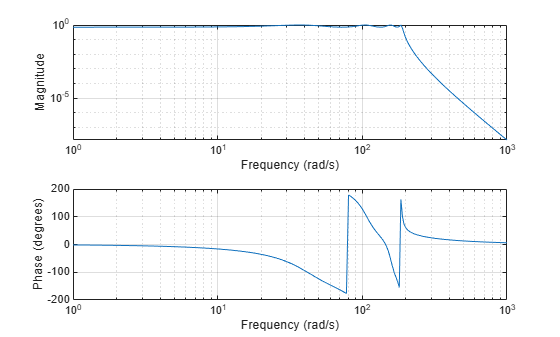# lp2lp

Change cutoff frequency for lowpass analog filter

## Syntax

``[bt,at] = lp2lp(b,a,Wo)``
``[At,Bt,Ct,Dt] = lp2lp(A,B,C,D,Wo)``

## Description

example

````[bt,at] = lp2lp(b,a,Wo)` transforms an analog lowpass filter prototype given by polynomial coefficients (specified by row vectors `b` and `a`) into a lowpass filter with cutoff angular frequency `Wo`. The input system must be an analog filter prototype.```
````[At,Bt,Ct,Dt] = lp2lp(A,B,C,D,Wo)` converts the continuous-time state-space lowpass filter prototype (specified by matrices `A`, `B`, `C`, and `D`) to a lowpass filter with cutoff angular frequency `Wo`. The input system must be an analog filter prototype.```

## Examples

collapse all

Design an 8th-order Chebyshev Type I analog lowpass filter prototype with 3 dB of ripple in the passband.

`[z,p,k] = cheb1ap(8,3);`

Convert the prototype to transfer function form and display its magnitude and frequency responses.

```[b,a] = zp2tf(z,p,k); freqs(b,a)```Transform the prototype to a lowpass filter with a cutoff frequency of 30 Hz. Specify the cutoff frequency in rad/s. Display the magnitude and frequency responses of the transformed filter.

```Wo = 2*pi*30; [bt,at] = lp2lp(b,a,Wo); freqs(bt,at)```## Input Arguments

collapse all

Prototype numerator and denominator coefficients, specified as row vectors. `b` and `a` specify the coefficients of the numerator and denominator of the prototype in descending powers of s:

`$\frac{B\left(s\right)}{A\left(s\right)}=\frac{b\left(1\right){s}^{n}+\cdots +b\left(n\right)s+b\left(n+1\right)}{a\left(1\right){s}^{m}+\cdots +a\left(m\right)s+a\left(m+1\right)}$`

Data Types: `single` | `double`

Prototype state-space representation, specified as matrices. The state-space matrices relate the state vector x, the input u, and the output y through

`$\begin{array}{l}\stackrel{˙}{x}=Ax+Bu\\ y=Cx+Du\end{array}$`

Data Types: `single` | `double`

Cutoff angular frequency, specified as a scalar. Express `Wo` in units of rad/s.

Data Types: `single` | `double`

## Output Arguments

collapse all

Transformed numerator and denominator coefficients, returned as row vectors.

Transformed state-space representation, returned as matrices.

## Algorithms

`lp2lp` transforms an analog lowpass filter prototype with a cutoff angular frequency of 1 rad/s into a lowpass filter with any specified cutoff angular frequency. The transformation is one step in the digital filter design process for the `butter`, `cheby1`, `cheby2`, and `ellip` functions.

`lp2lp` is a highly accurate state-space formulation of the classic analog filter frequency transformation. If a lowpass filter has cutoff angular frequency ω0, the standard s-domain transformation is

`$s=p/{\omega }_{0}$`

The state-space version of this transformation is

`$At={\omega }_{0}\cdot A$`
`$Bt={\omega }_{0}\cdot B$`
`$Ct=C$`
`$Dt=D$`

The `lp2lp` function can perform the transformation on two different linear system representations: transfer function form and state-space form. See `lp2bp` for a derivation of the bandpass version of this transformation.